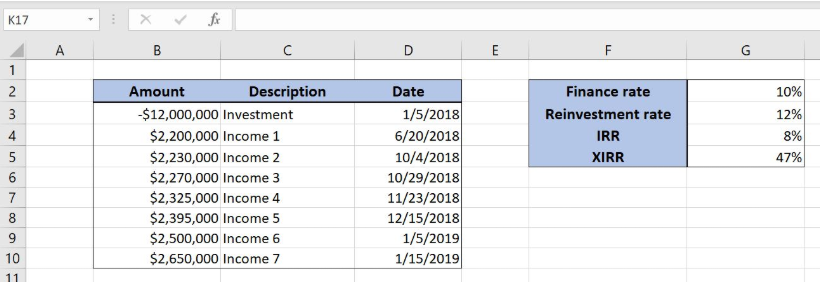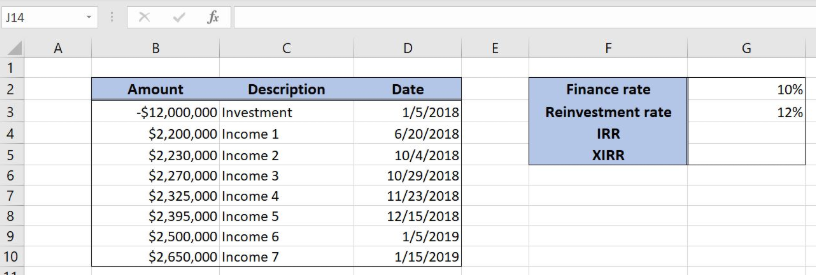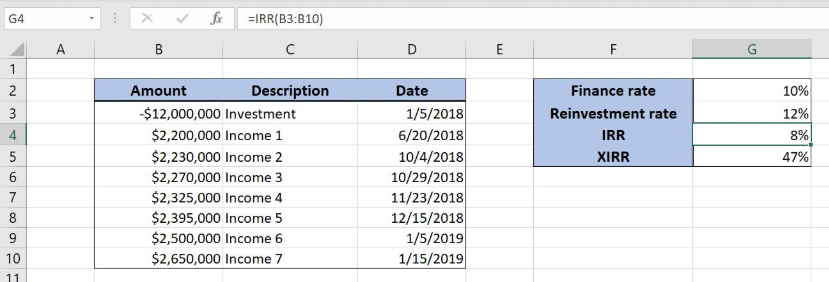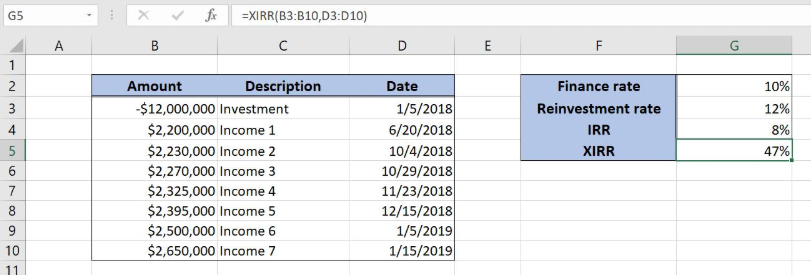Get instant live expert help with Excel or Google Sheets“My Excelchat expert helped me in less than 20 minutes, saving me what would have been 5 hours of work!”

#### Post your problem and you’ll get expert help in seconds.

Your message must be at least 40 characters
Our professional experts are available now. Your privacy is guaranteed.

# What is the Difference Between the IRR and XIRR Functions in Excel?

Excel allows a user to get an internal rate of return of an investment with returns occurring at regular or irregular intervals. If we want to use regular intervals we should use the IRR function. If we want to calculate for irregular intervals, then we need the XIRR function. This step by step tutorial will assist all levels of Excel users in learning what is the difference between the IRR and XIRR functions in Excel.Figure 1. The result of the IRR, XIRR and MIRR functions

## Syntax of the IRR Formula

The generic formula for the IRR function is:

`=IRR (values, [guess])`

The parameter of the IRR function is:

• values – a range of cells containing values, including initial investment and incomes. The investment must have a negative sign, as it is a cost
• [guess] – an estimated value for the expected IRR. This parameter is non-mandatory. If it’s omitted, the function will take a default value of 0.1 (=10%).

## Syntax of the XIRR Formula

The generic formula for the XIRR function is:

`=XIRR (values, dates, [guess])`

The parameter of the XIRR function is:

• values – a range of cells containing values, including initial investment and incomes. The investment must have a negative sign, as it is a cost
• dates – a range of dates corresponding to values. All dates must be in chronological order
• [guess] – an estimated value for the expected XIRR. This parameter is non-mandatory. If it’s omitted, the function will take a default value of 0.1 (=10%).

## Setting up Our Data for the Functions

Let’s look at the structure of the data we will use. In column B (“Amount”), we have values including initial investment and yearly incomes. In column C (“Description”) we have a description of every amount. In column D (“Date”), we have dates. In In the cell G4, G5 and G6, we want to get the IRR, XIRR and MIRR respectively.Figure 2. Data that we will use in the examples

## Get an IRR of Values Using the IRR Function

In our example, we want to get the IRR of the values in the range B3:B10. The result will be in the cell E3.

The formula looks like:

`=IRR(B3:B10)`

The parameter values is the range B3:B10.

To apply the IRR function, we need to follow these steps:

• Select cell G4 and click on it
• Insert the formula: `=IRR(B3:B10)`
• Press enter.Figure 3. Using the IRR function to get the internal rate of the investment

Finally, the result in the cell G4 is 8%, which is the internal rate of the investment using the IRR function.

## Get an XIRR of Values Using the XIRR Function

In our example, we want to get the XIRR of the values in the range B3:B10. The result will be in the cell E3.

The formula looks like:

`=XIRR(B3:B10, D3:D10)`

The parameter values is the range B3:B10 and the parameter dates is D3:D10.

To apply the XIRR function, we need to follow these steps:

• Select cell G5 and click on it
• Insert the formula: `=XIRR(B3:B10, D3:D10)`
• Press enter.Figure 4. Using the XIRR function to get the internal rate of the investment

Finally, the result in the cell G5 is 47%, which is the internal rate of the investment using the XIRR function.

Most of the time, the problem you will need to solve will be more complex than a simple application of a formula or function. If you want to save hours of research and frustration, try our live Excelchat service! Our Excel Experts are available 24/7 to answer any Excel question you may have. We guarantee a connection within 30 seconds and a customized solution within 20 minutes.